By using only two resistance coils-singly, in series, or in parallel one should be able to obtain resistances of 3, 4, 12, and 16 ohms. The separate resistances of the coil are :

(1) 3 and 4

(2) 4 and 12

(3) 12 and 16

(4) 16 and 3

Concept Questions :-

Combination of resistors
High Yielding Test Series + Question Bank - NEET 2020

Difficulty Level:

In the given circuit, the voltmeter records 5 volts. The resistance of the voltmeter in ohms is :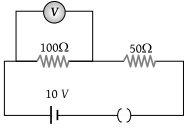(1) 200

(2) 100

(3) 10

(4) 50

Concept Questions :-

Kirchoff's voltage law
High Yielding Test Series + Question Bank - NEET 2020

Difficulty Level:

In the adjoining circuit, the battery E1 has an e.m.f. of 12 volts and zero internal resistance while the battery E has an e.m.f. of 2 volts. If the galvanometer G reads zero, then the value of the resistance X in ohm is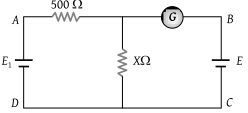(1) 10

(2) 100

(3) 500

(4) 200

Concept Questions :-

Grouping of cells
High Yielding Test Series + Question Bank - NEET 2020

Difficulty Level:

The magnitude and direction of the current in the circuit shown will be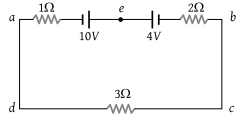(1) $\frac{7}{3}$A from a to b through e

(2) $\frac{7}{3}$A from b to a through e

(3) 1A from b to a through e

(4) 1A from a to b through e

Concept Questions :-

Grouping of cells
High Yielding Test Series + Question Bank - NEET 2020

Difficulty Level:

The e.m.f. of a cell is E volts and internal resistance is r ohm. The resistance in external circuit is also r ohm. The p.d. across the cell will be

(1) E/2

(2) 2E

(3) 4E

(4) E/4

Concept Questions :-

Emf and terminal voltage
High Yielding Test Series + Question Bank - NEET 2020

Difficulty Level:

Kirchhoff's first law i.e. $\Sigma i=0$ at a junction is based on the law of conservation of :

(1) Charge

(2) Energy

(3) Momentum

(4) Angular momentum

Concept Questions :-

Kirchoff's current law
High Yielding Test Series + Question Bank - NEET 2020

Difficulty Level:

The figure below shows currents in a part of electric circuit. The current i is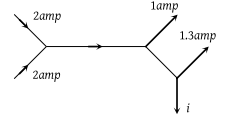(1) 1.7 amp

(2) 3.7 amp

(3) 1.3 amp

(4) 1 amp

Concept Questions :-

Kirchoff's current law
High Yielding Test Series + Question Bank - NEET 2020

Difficulty Level:

In the circuit shown, A and V are ideal ammeter and voltmeter respectively. Reading of the voltmeter will be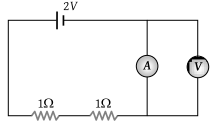(1) 2 V

(2) 1 V

(3) 0.5 V

(4) Zero

Concept Questions :-

Kirchoff's voltage law
High Yielding Test Series + Question Bank - NEET 2020

Difficulty Level:

The terminal potential difference of a cell when short-circuited is (E = E.M.F. of the cell)

(1) E

(2) E/2

(3) Zero

(4) E/3

Concept Questions :-

Emf and terminal voltage
High Yielding Test Series + Question Bank - NEET 2020

Difficulty Level:

The potential difference in open circuit for a cell is 2.2 volts. When a 4-ohm resistor is connected between its two electrodes the potential difference becomes 2 volts. The internal resistance of the cell will be :

(1) 1 ohm

(2) 0.2 ohm

(3) 2.5 ohm

(4) 0.4 ohm

Concept Questions :-

Emf and terminal voltage